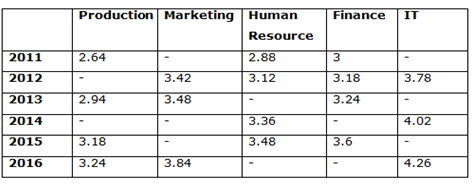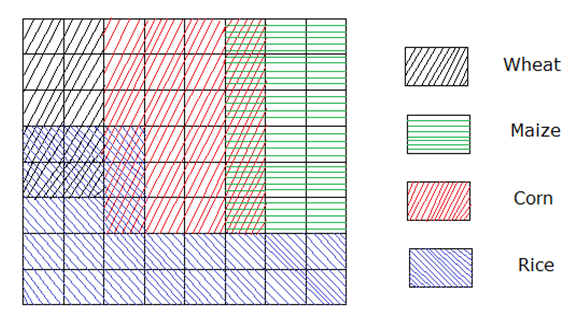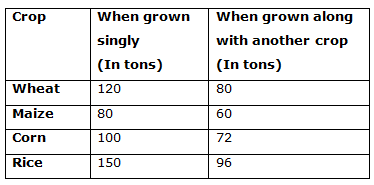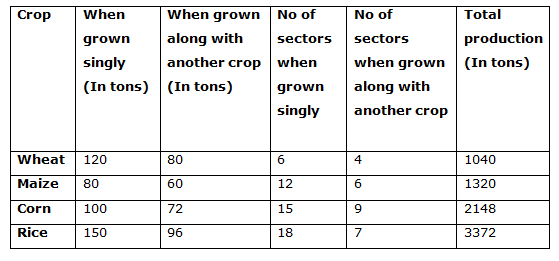# SBI PO Quantitative Aptitude Questions 2019 (Day-13) High Level New Pattern

SBI PO 2019 Notification is about to come and it is the most awaited exam among the aspirants. We all know that new pattern questions are introducing every year in the SBI PO exam. Further, the questions are getting tougher and beyond the level of the candidate’s expectations.

Our IBPS Guide is providing High-Level New Pattern Quantitative Aptitude Questions for SBI PO 2019 so the aspirants can practice it on a daily basis. These questions are framed by our skilled experts after understanding your needs thoroughly. Aspirants can practice these high-level questions daily to familiarize with the exact exam pattern. We wish that your rigorous preparation leads you to a successful target of becoming SBI PO.

[WpProQuiz 4989]

### Click Here for SBI PO Pre 2019 High-Quality Mocks Exactly on SBI Standard

Directions (1 – 5): Study the following information carefully and answer the given questions:

The following table shows the annual salary (In lakhs) of each employee in different departments of a certain company over the years. Some values are missing here.1) Find the difference between the total monthly salary of each employees in all the given departments together in the year 2011 to that in the year 2015, if the annual salary of each IT employee in the year 2011 is 3.72 lakhs and the sum of the monthly salary of each marketing employee in the year 2011 and 2012 is Rs. 56500 and the sum of the annual salary of each Marketing and IT employees in the year 2015 is 7.98 lakhs and the annual salary of each marketing employees in the year 2015 is Rs. 42000 less than the annual salary of each IT employees in the year 2015?

a) Rs. 26000

b) Rs. 42000

c) Rs. 22000

d) Rs. 45000

e) None of these

2) Find the average annual salary of each marketing employees in all the given years together, if the annual salary of each marketing employees in the year 2014 is same as the annual salary of each Finance department employees in the year 2015 and the average annual salary of each marketing employees in the year 2011 and 2015 is 3.57 lakhs?

a) 3.58 lakhs

b) 3.32 lakhs

c) 3.94 lakhs

d) 4.16 lakhs

e) None of these

3) If there were 40 employees in Human resource department in the year 2012 and 85 employees in the same department in the year 2016, then find the total amount spent on both the years together for the salaries of total employees in Human resource department, while the monthly salary of each Human resource development employees in the year 2015 and 2016 together is Rs. 58500?

a) 378.5 lakhs

b) 434.2 lakhs

c) 391.6 lakhs

d) 425.7 lakhs

e) None of these

4) Find the ratio between the total monthly salary of each production department employees to that of each IT employees in all the given years together, if the monthly salary of each production department employee in the year 2012 is Rs. 24000 and the annual salary of each production department employee in the year 2014 is Rs. 12000 more than the annual salary of each production department employee in the year 2012 and the sum of the monthly salary of each IT employees in the year 2011 and 2013 together is Rs. 64000 and the annual salary of each IT employee in the year 2015 is 4.2 lakhs?

a) 157: 223

b) 298: 399

c) 113: 175

d) 52: 67

e) None of these

5) The annual salary of each employee in the year 2012 in all the given department together is approximately what percentage of the annual salary of each employee in the year 2015 in all the given department together, if the sum of annual salary of each employee in Marketing and IT department in the year 2015 is 7.98 lakhs and the monthly salary of each employee in production department in the year 2012 is Rs. 24000?

a) 110 %

b) 75 %

c) 90 %

d) 135 %

e) 60 %

Directions (6 – 10): Study the following information carefully and answer the given questions.

Magi has a large farm land in the shape of a square that is further divided into 64 smaller squares called sectors. Each sector is cultivated with either one or two crops. The pattern of crop cultivation distributed over his land in the year 2015 is as shown below.The capacity of each sector to produce a particular crop depends on whether one or two crops are being grown in that sector. The table below gives the production capacity of a sector for each of the 4 crops.6) Total production of Wheat in the year 2015 is approximately what percentage more/less than the total production of Rice in the same year?

a) 55 % less

b) 55 % more

c) 69 % less

d) 69 % more

e) 82 % more

7) If 50 % of the area cultivated with only rice is now used to cultivate wheat as well, then what is the new ratio of the production of rice to the total production of wheat?

a) 1441: 881

b) 1521: 883

c) 1443: 880

d) 1483: 691

e) None of these

8) If the cost price of corn and Maize is Rs. 1200 per ton and Rs. 1540 per ton

Quantity I: Find the total selling price of corn if it is sold at 15% profit?

Quantity II: Find the total selling price of Maize if it is sold at 25% profit?

a) Quantity I < Quantity II

b) Quantity I ≤ Quantity II

c) Quantity I > Quantity II

d) Quantity I ≥ Quantity II

e) Quantity I = Quantity II (or) Relationship cannot be determined

9) Which crop recorded lowest production when grown along with another crop?

a) Rice

b) Corn

c) Maize

d) Wheat

e) Both (a) and (c)

10) In 2016, the production of Wheat, Corn, Rice and Maize is increased by 60%, 40%, 25% and 50% from previous year respectively, then find the total production of all the crop together

a) 12547 tons

b) 11255 tons

c) 14523 tons

d) 13459 tons

e) None of these

Direction (1 – 5):

The annual salary of each IT employee in the year 2011 = 3.72 lakhs

The sum of the monthly salary of each marketing employee in the year 2011 and 2012 = Rs. 56500

The monthly salary of each marketing employee in the year 2011

= > 56500 – (342000/12)

= > 56500 – 28500 = Rs. 28000

The total monthly salary of each employee in all the given departments together in the year 2011

= > (264000/12) + 28000 + (288000/12) + (300000/12) + (372000/12)

= > 22000 + 28000 + 24000 + 25000 + 31000 = Rs. 130000

The sum of the annual salary of each Marketing and IT employees in the year 2015 = 7.98 lakhs

IT + Marketing = 798000 – (1)

The annual salary of each marketing employees in the year 2015 = The annual salary of IT employees in the year 2015 – 42000

IT – Marketing = 42000 – (2)

By solving the equation (1) and (2), we get,

IT = 4.2 lakhs, Marketing = 3.78 lakhs

The total monthly salary of each employee in all the given departments together in the year 2015

= > (318000/12) + (378000/12) + (348000/12) + (360000/12) + (420000/12)

= > 26500 + 31500 + 29000 + 30000 + 35000 = Rs. 152000

Required difference = 152000 – 130000 = Rs. 22000

The annual salary of each marketing employees in the year 2014 = The annual salary of each Finance department employees in the year 2015

The annual salary of each marketing employees in the year 2014 = 3.6 lakhs

The average annual salary of each marketing employees in the year 2011 and 2015

= > 3.57 lakhs

The total annual salary of each marketing employees in the year 2011 and 2015

= > 3.57*2 = 7.14 lakhs

The average annual salary of each marketing employees in all the given years together

= > (7.14 + 3.42 + 3.48 + 3.6 + 3.84)/6

= > 21.48/6 = 3.58 lakhs

The monthly salary of each Human resource development employees in the year 2015 and 2016 together = Rs. 58500

The monthly salary of each Human resource development employee in the year 2016 = > 58500 – (348000/12)

= > 58500 – 29000 = Rs. 29500

The annual salary of each Human resource development employee in the year 2016

= > 29500*12 = Rs. 354000

The total amount spent for the salaries of total employees in Human resource department in the year 2012 and 2016 together

= > (3.12*40) + (3.54*85)

= > 124.8 + 300.9 = 425.7 lakhs

The monthly salary of each production department employee in the year 2012

= Rs. 24000

The annual salary of each production department employee in the year 2014 = Rs. 12000 + The annual salary of each production department employee in the year 2012

= 288000+12000 = 300000

So, the monthly salary of each production department employee in the year 2014 = 300000/12 = Rs. 25000

The total monthly salary of each production department employees in all the given years together

= > (264000/12) + 24000 + (294000/12) + 25000 + (318000/12) + (324000/12)

= > 22000 + 24000 + 24500 + 25000 + 26500 + 27000

= > Rs. 149000

The sum of the monthly salary of each IT employees in the year 2011 and 2013 together = Rs. 64000

The annual salary of each IT employee in the year 2015 = 4.2 lakhs

The salary of each IT employees in the year 2015 = 420000/12 = Rs. 35000

The total monthly salary of each IT employees in all the given years together

= > 64000 + (378000/12) + (402000/12) + 35000 + (426000/12)

= > 64000 + 31500 + 33500 + 35000 + 35500 = Rs. 199500

Required ratio = 149000: 199500 = 298: 399

The monthly salary of each employee in production department in the year 2012 = > Rs. 24000

The annual salary of each employee in the year 2012 in all the given department together

= > (24000*12) + 342000 + 312000 + 318000 + 378000

= > 1638000 = 16.38 lakhs

The sum of annual salary of each employee in Marketing and IT department in the year 2015 = 7.98 lakhs

The annual salary of each employee in the year 2015 in all the given department together

= > 3.18 + 7.98 + 3.48 + 3.6 = 18.24 lakhs

Required % = (16.38/18.24)*100 = 90 %

Directions (6 – 10):Required percentage = [(3372 – 1040)/3372]*100 = 69 % less

50% of the area cultivated with only rice = 18 * 50/100 = 9 sectors

No of sectors cultivated only rice = 9 sectors

No of sectors cultivated rice along with another crop = 9 + 7 = 16 sectors

Total production = (9*150) + (16*96) = 2886 tons

No of sectors cultivated only Wheat = 6 sectors

No of sectors cultivated wheat along with another crop = 4 + 9 = 13 sectors

Total production = (6*120) + (13*80) = 1760

Required ratio = 2886: 1760 = 1443: 880

Total cost price of corn = (1200*2148) = 25.776 lakhs

Total cost price of Maize = (1540*1320) = 20.328 lakhs

Quantity I:

Total selling price = 25.776 lakhs * (115/100) = 29.6424 lakhs

Quantity II:

Total selling price = 20.328 * (125/100) = 25.41 lakhs

Quantity I > Quantity II

Total Wheat production = 80 * 4 = 320 tons

Total Maize production = 60 * 6 = 360 tons

Total Corn production = 72 * 9 = 648 tons

Total Rice production = 96 * 7 = 672 tons

Wheat crop recorded lowest production when grown along with another crop.

In the year 2016:

Total Wheat production = 1040 * 160/100 = 1664 tons

Total Corn production = 1320 * 140/100 = 1848 tons

Total Rice production = 2148 * 125/100 = 2685 tons

Total Maize production = 3372 * 150/100 = 5058 tons

Total production in the year 2016 = (1664 + 1848 + 2685 + 5058) tons

= 11255 tons

### Click Here for SBI PO Pre 2019 High-Quality Mocks Exactly on SBI Standard